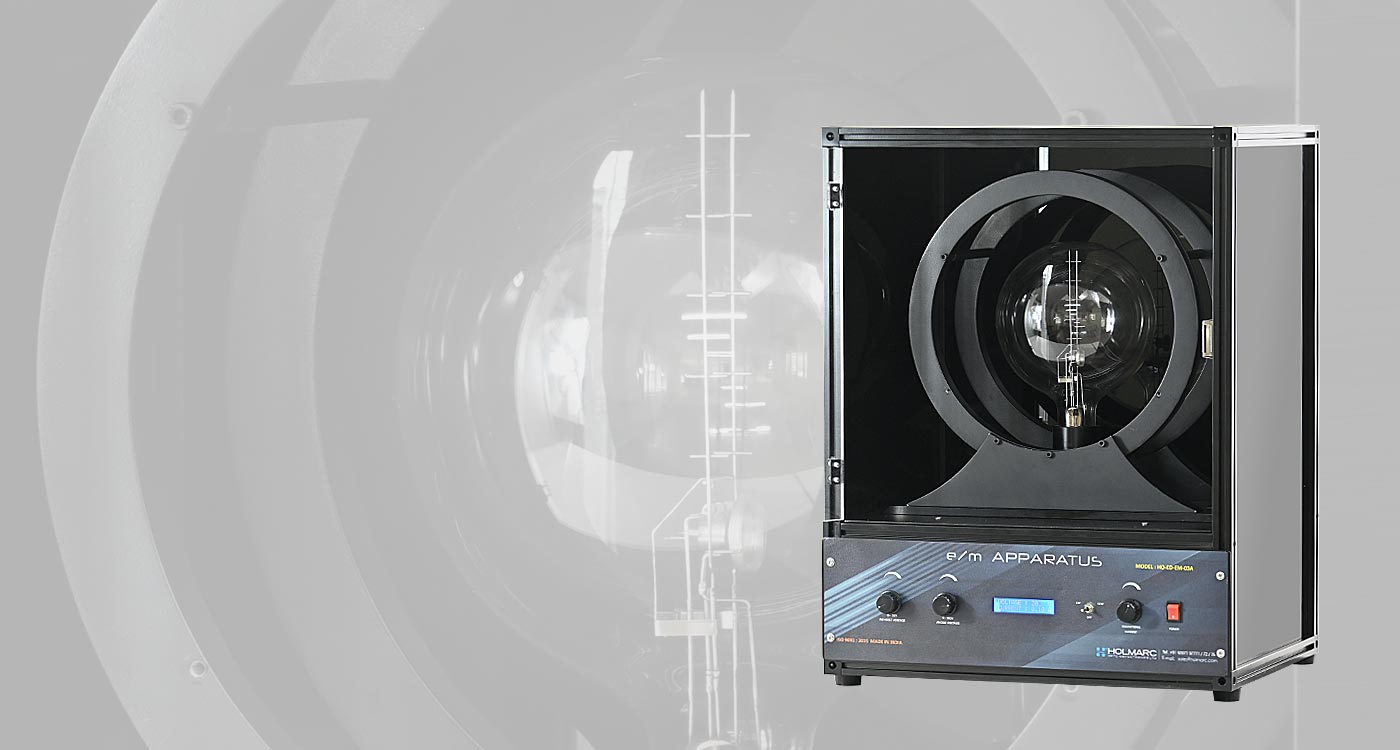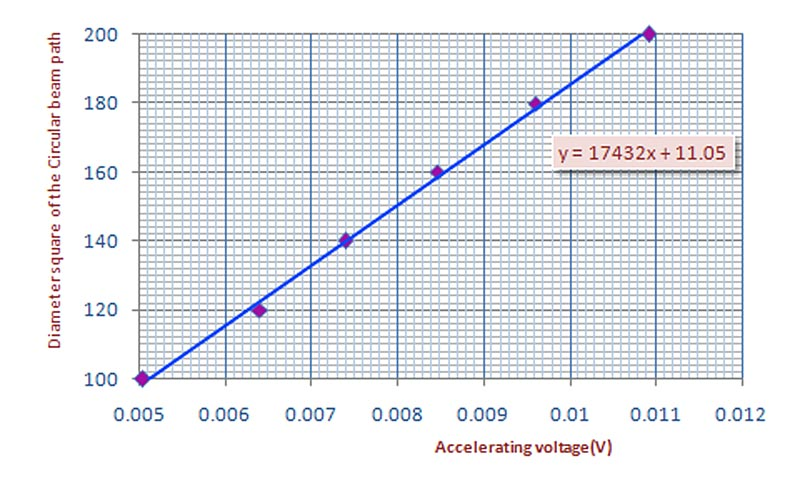e/m Experiment Apparatus

(Thomson’s method)

Model: HO-ED-EM-03

Holmarc's e/m apparatus (Model No: HO-ED-EM-03) is designed for the measurement of the charge to mass ratio e/m, of the electron. This equipment also facilitates the demonstration of effects of electric and magnetic fields on a moving charged particle. It consists of a fine beam electron tube known as e/m tube, a pair of Helmholtz coils and the electronic control box. The e/m tube consists of a filament as cathode, an accelerating anode and a pair of deflection plates. The heater heats the cathode, which emits electrons. The electrons are accelerated by the potential applied between the cathode and the anode.

The grid is held positive with respect to the cathode and negative with respect to the anode. The tube is filled with helium at low pressure. The electron tube is placed between a pair of Helmholtz coils.

The electronic control unit provides the energy requirements for the tube and Helmholtz coil. The Helmholtz coil is a pair of coils separated by a distance of the coil radius. The e/m tube generates a fine electron beam by applying accelerating voltage. Electron collisions with the gas molecules excite the gas molecules and produce light emission in a cylindrical sheath around the electron beam. As the excited molecules relax, the electron paths become visible.

This e/m apparatus is also used for demonstration of electron beam deflection using the deflection plates. When we apply current to the Helmholtz coil, uniform magnetic field is generated and as a result, the fine electron beam changes its path to circular. By measuring the accelerating potential (V), the current through the Helmholtz coils (I) and the radius of the circular electron beam (r) we can find out the electron-mass ratio.

Experiment Examples

To measure the Charge to Mass ratio of Electron (e/m)

e/m   =   [  (  125 a2  /  128 π2 N2  )  x  1014  ]  (  V  /  I2 D2  )

Where

V   -   Accelerating Potential

a   -   Radius Of The Helmholtz Coils

N   -   Number Of Turns On Each Helmholtz Coil

I   -   Current Through The Helmholtz Coils

D   -   Diameter Of The Electron Beam Path

In our Helmholtz coil number of turns on each coil N = 140 and the radius a = 13.5 cm

Specification

Accelerating voltage   :   0 - 250 V DC

Coil current   :   0.5 - 2.5 A, reversible

Deflection plate voltage   :   50-250 V, reversible

Display   :   Two row LCD display

Power input   :   230 VAC 50 HzFeatures

Excellent for accurate measurements of charge-to-mass ratio

Clear observation of circular electron beam path

Change in direction of the coil current possible
Related Topics

e/m Ratio

Helmholtz coil

Lorentz force

Cathode

Anode

|

©2018 Holmarc Opto-Mechatronics (P) Ltd. All rights reserved.